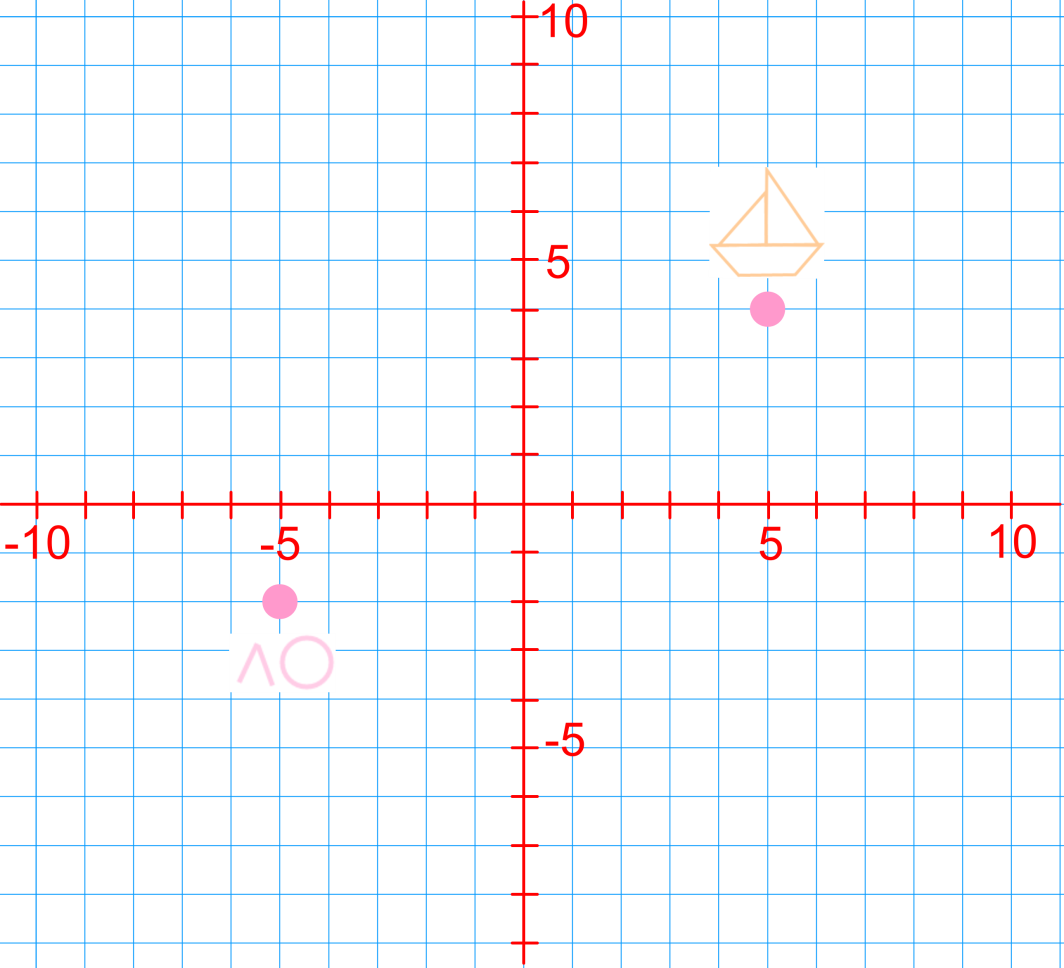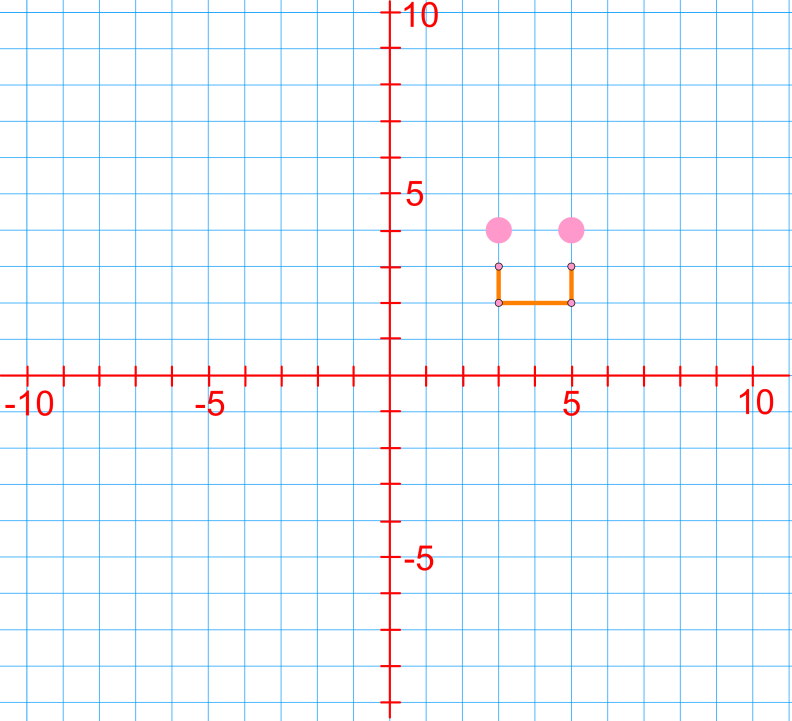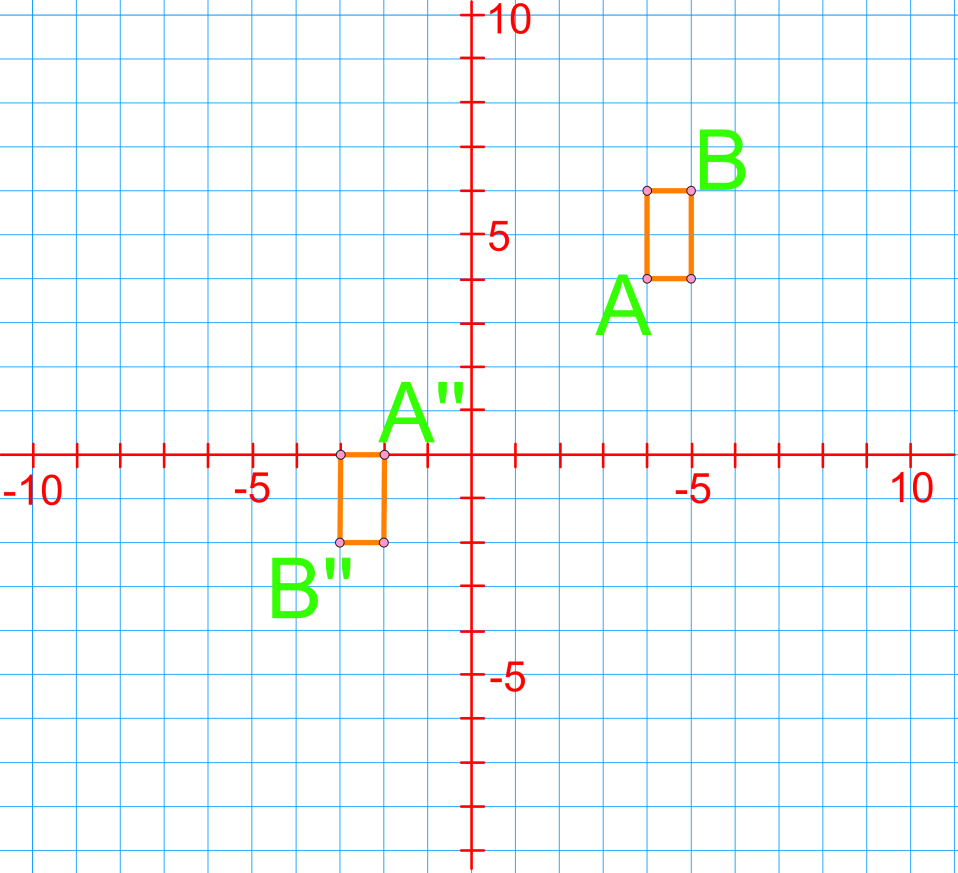# Horizontal and vertical distances

### Horizontal and vertical distances

In this section, we will be showing and describing transformations of given figures on coordinate planes. In the previous section, we used only one type of transformation for each question. In contrast, in this section, we will be using several types of transformations for each question. For example, we will be given a figure on a coordinate plane and asked to describe what combination of translations, reflections and rotations would need to occur to move this figure from one location to another on the plane.

#### Lessons

• Introduction
Types of transformations:
What are translations, reflections and rotations?

• 1.
a)
Use translations, reflections and rotations to get the swimmer to the boat.b)
Describe the total horizontal and vertical distance the swimmer traveled from start to finish.• 2.
Reflect this shape in the x-axis and then translate it 3 units horizontally left and 1 unit vertically down.• 3.
Describe the movement of Vertex A and Vertex B.